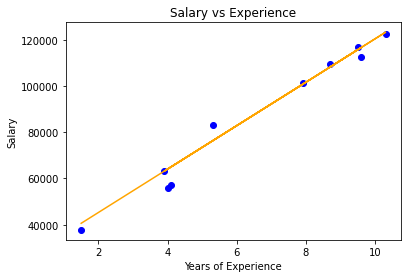Open in App
Not now

# Linear Regression Implementation From Scratch using Python

• Difficulty Level : Medium
• Last Updated : 01 Oct, 2020

Linear Regression is a supervised learning algorithm which is both a statistical and a machine learning algorithm. It is used to predict the real-valued output y based on the given input value x. It depicts the relationship between the dependent variable y and the independent variables xi  ( or features ).  The hypothetical function used for prediction is represented by h( x ).

  h( x ) = w * x + b

here, b is the bias.
x represents the feature vector
w represents the weight vector.


Linear regression with one variable is also called univariant linear regression.  After initializing the weight vector, we can find the weight vector to best fit the model by ordinary least squares method or gradient descent learning.

Mathematical Intuition: The cost function (or loss function) is used to measure the performance of a machine learning model or quantifies the error between the expected values and the values predicted by our hypothetical function. The cost function for Linear Regression is represented by J.Here, m is the total number of training examples in the dataset.
y(i) represents the value of target variable for ith training example.


So, our objective is to minimize the cost function J (or improve the performance of our machine learning model). To do this, we have to find the weights at which J is minimum.  One such algorithm which can be used to minimize any differentiable function is Gradient Descent. It is a first-order iterative optimizing algorithm that takes us to a minimum of a function.

Pseudo Code:

2. Keep changing w to reduce J( w ) until we hopefully end up at a minimum.

Algorithm:

repeat until convergence  {
tmpi = wi - alpha * dwi
wi = tmpi
}
where alpha is the learning rate.


#### Implementation:

It has 2 columns — “YearsExperience” and “Salary” for 30 employees in a company. So in this, we will train a Linear Regression model to learn the correlation between the number of years of experience of each employee and their respective salary. Once the model is trained, we will be able to predict the salary of an employee on the basis of his years of experience.

## Python3

 # Importing libraries  import numpy as np  import pandas as pd  from sklearn.model_selection import train_test_split  import matplotlib.pyplot as plt  # Linear Regression  class LinearRegression() :          def __init__( self, learning_rate, iterations ) :                  self.learning_rate = learning_rate                  self.iterations = iterations              # Function for model training                  def fit( self, X, Y ) :                  # no_of_training_examples, no_of_features                  self.m, self.n = X.shape                  # weight initialization                  self.W = np.zeros( self.n )                  self.b = 0                  self.X = X                  self.Y = Y                            # gradient descent learning                          for i in range( self.iterations ) :                          self.update_weights()                      return self          # Helper function to update weights in gradient descent          def update_weights( self ) :                     Y_pred = self.predict( self.X )                  # calculate gradients                dW = - ( 2 * ( self.X.T ).dot( self.Y - Y_pred )  ) / self.m               db = - 2 * np.sum( self.Y - Y_pred ) / self.m                   # update weights              self.W = self.W - self.learning_rate * dW              self.b = self.b - self.learning_rate * db                  return self          # Hypothetical function  h( x )           def predict( self, X ) :              return X.dot( self.W ) + self.b       # driver code  def main() :          # Importing dataset          df = pd.read_csv( "salary_data.csv" )      X = df.iloc[:,:-1].values      Y = df.iloc[:,1].values          # Splitting dataset into train and test set      X_train, X_test, Y_train, Y_test = train_test_split(       X, Y, test_size = 1/3, random_state = 0 )          # Model training          model = LinearRegression( iterations = 1000, learning_rate = 0.01 )      model.fit( X_train, Y_train )          # Prediction on test set      Y_pred = model.predict( X_test )          print( "Predicted values ", np.round( Y_pred[:3], 2 ) )           print( "Real values      ", Y_test[:3] )          print( "Trained W        ", round( model.W, 2 ) )          print( "Trained b        ", round( model.b, 2 ) )          # Visualization on test set           plt.scatter( X_test, Y_test, color = 'blue' )          plt.plot( X_test, Y_pred, color = 'orange' )          plt.title( 'Salary vs Experience' )          plt.xlabel( 'Years of Experience' )          plt.ylabel( 'Salary' )          plt.show()     if __name__ == "__main__" :           main()

#### Output:

Predicted values  [ 40594.69 123305.18  65031.88]
Real values       [ 37731 122391  57081]
Trained W         9398.92
Trained b         26496.31Linear Regression Visualization

My Personal Notes arrow_drop_up